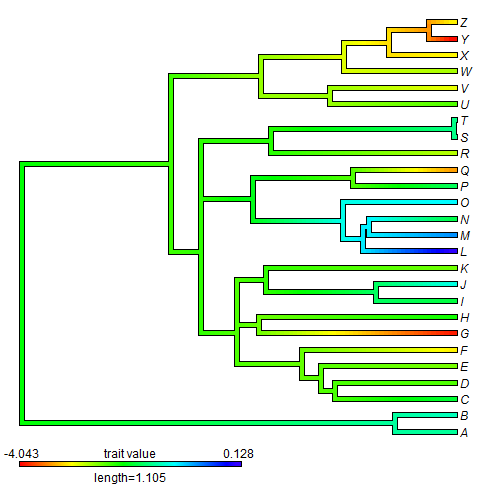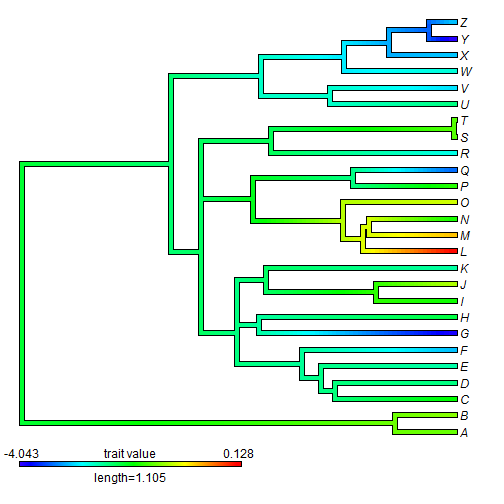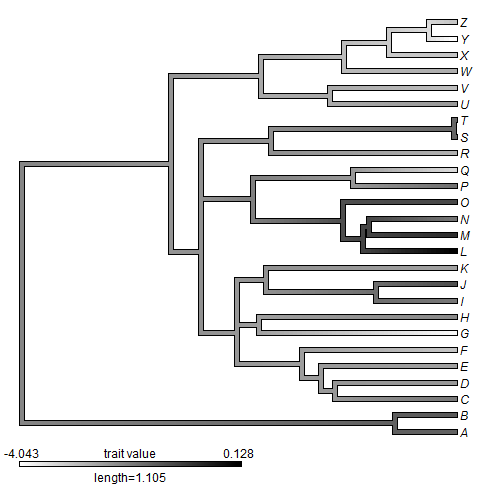## Sunday, December 7, 2014

### Inverting the color map on an object of class "contMap" or "densityMap"

In response to a recent user comment I have just posted code to automatically flip or invert the color map in an object of class `"contMap"` or `"densityMap"`. The function is very simple, as follows:

``````setMap<-function(x,...){
if(hasArg(invert)) invert<-list(...)\$invert
else invert<-FALSE
n<-length(x\$cols)
if(invert) x\$cols<-setNames(rev(x\$cols),names(x\$cols))
else x\$cols[1:n]<-colorRampPalette(...)(n)
x
}
``````

Here is how it works:

``````library(phytools)
``````
``````## Loading required package: ape
##
## Attaching package: 'phytools'
##
## The following object is masked _by_ '.GlobalEnv':
##
##     setMap
``````
``````tree<-pbtree(n=26,tip.label=LETTERS)
x<-fastBM(tree)
obj<-contMap(tree,x)
````````````plot(setMap(obj,invert=TRUE))
``````Remember, `setMap` can also be used to change the map arbitrarily, such as in:

``````obj<-setMap(obj,colors=c("white","black"))
plot(obj)
``````That's it.

1.Liam,
Thanks this is a super cool to plot continuous characters.

Any idea why the "type" argument isn't passed on through the setmap code?

For instance, in the above:

obj<-contMap(tree,x,type="fan") - works great produces circular phylogram

But then:

plot(setMap(obj,invert=TRUE)) - reverts back to square phylogram (with inverted colors!)

Actually, any color changes don't seem to be passed on to a type "fan". Any idea whassa going' on?

Ryan

1.Nevermind, I just realized that you can set all of your parameters after during the "plot" call, I had no idea that "plot" would recognize these:

plot(setMap(obj,invert=TRUE),type="fan",fsize=0.5,lwd=2)

COOLIO!

2.Glad to hear you figured it out! - Liam

2.I am running a tree with 211 tips and 4 discrete states. I keep getting a script out of bounds error when I try to initiate make.simmap. If I reduce the data files to 20 tips as a test everything works fine. This suggests the files is too big, right? How do I get around that?

3.Sorry for the "unkown, my name is Lee Grismer

Note: due to the very large amount of spam, all comments are now automatically submitted for moderation.